### Home > PC > Chapter 2 > Lesson 2.3.4 > Problem2-119

2-119.
1. Use the triangle to answer the problems below. Homework Help ✎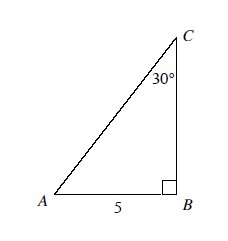1. Find the exact lengths of the missing sides.

2. Write and simplify the cosine ratio for ∠A.

3. Write and simplify the sine ratio for ∠A.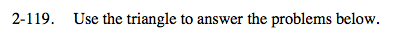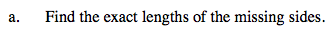Find the 3rd angle.
Then use the Law of Sines to find the other sides.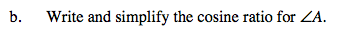$\text{Cos}A=\frac{\text{adjacent side}}{\text{hypotenuse}}$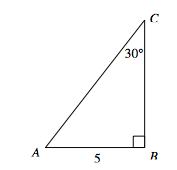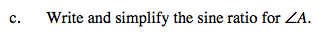$\text{Sin}A=\frac{\text{opposite side}}{\text{hypotenuse}}$